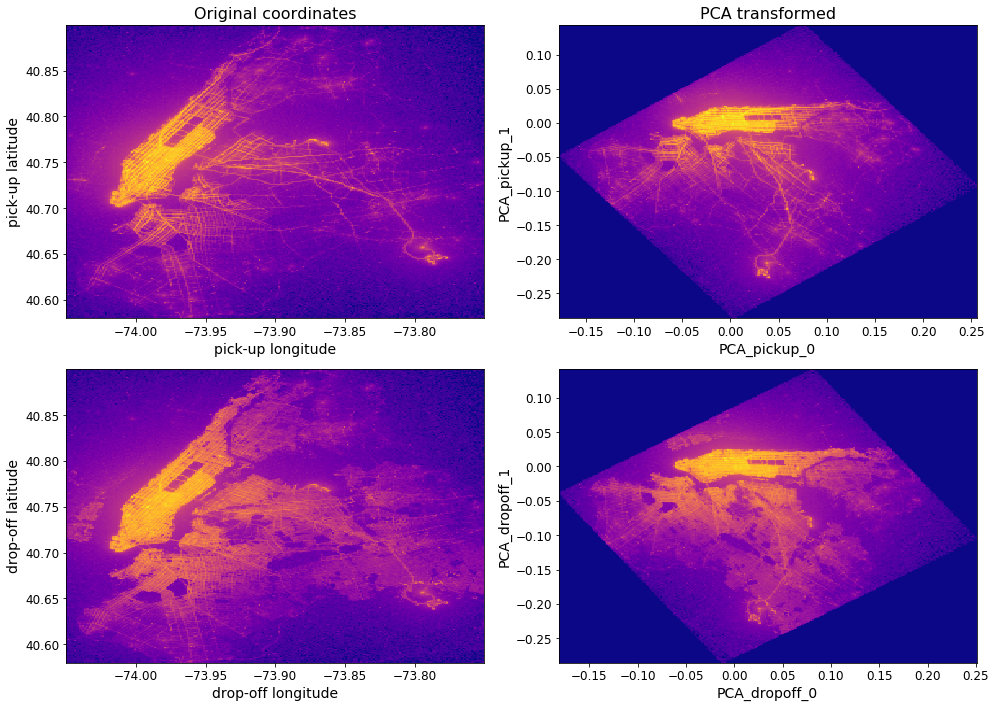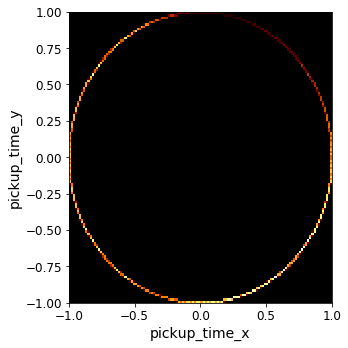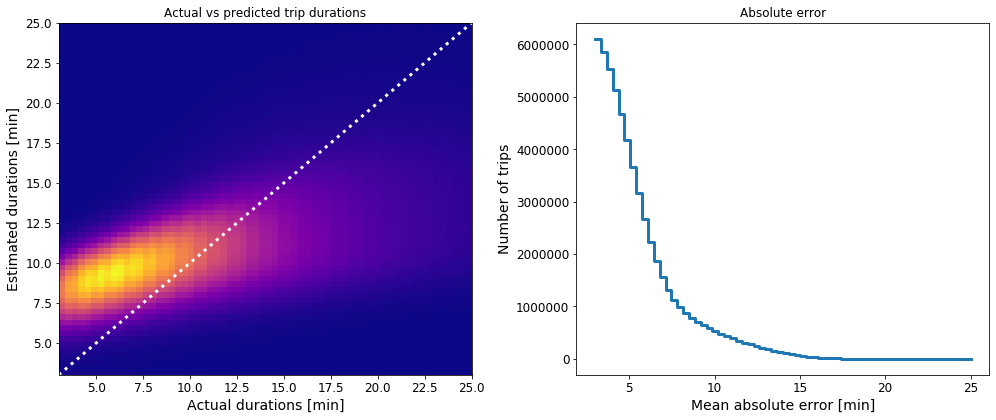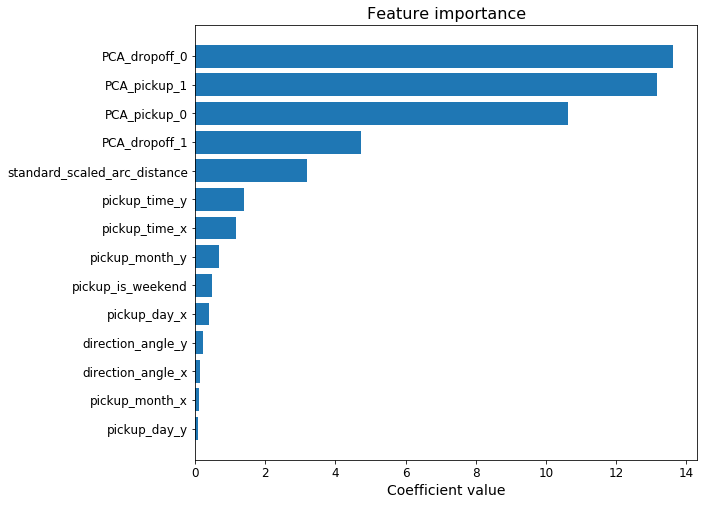# Machine Learning example on 1 billion samples: N. Y. CityCabs data¶

### Predict the likely duration of a taxi trip¶

In :
import vaex
import vaex.ml

import numpy as np

import pylab as plt

import warnings
warnings.filterwarnings("ignore")


### Adjusting matplotlib parameters¶

In :
SMALL_SIZE = 12
MEDIUM_SIZE = 14
BIGGER_SIZE = 16

plt.rc('font', size=SMALL_SIZE)          # controls default text sizes
plt.rc('axes', titlesize=SMALL_SIZE)     # fontsize of the axes title
plt.rc('axes', labelsize=MEDIUM_SIZE)    # fontsize of the x and y labels
plt.rc('xtick', labelsize=SMALL_SIZE)    # fontsize of the tick labels
plt.rc('ytick', labelsize=SMALL_SIZE)    # fontsize of the tick labels
plt.rc('legend', fontsize=SMALL_SIZE)    # legend fontsize
plt.rc('figure', titlesize=BIGGER_SIZE)  # fontsize of the figure title


• Can "read" the memmory mapped file that we have on disk in no time.
• Vaex can also read data stored on S3. The data is streamed on a need-to-have basis and is locally cached.
In :
# Check the file size on disk
!ls -l -h ./data/yellow_taxi_2009_2015_f32.hdf5

-rw-r--r--  1 jovan  staff   107G Jul  3  2019 ./data/yellow_taxi_2009_2015_f32.hdf5

In :
# Read in the data from disk
df = vaex.open('./data/yellow_taxi_2009_2015_f32.hdf5')

# # Alternatively, one can read in the data straight from AWS
# df = vaex.open('s3://vaex/taxi/yellow_taxi_2009_2015_f32.hdf5?anon=true')


Opening a DataFrame straight from S3 will lazily download the data. Given the large quantity of data used in this notebook, this might disrupt ones workflow. Thus it may be more convenient to simply pre-fetch the relevant portions of the data prior to doing any work.

In [ ]:
# One can also take a smaller subset of the data
# df = df[:10_000_000]

# used_columns = """dropoff_datetime pickup_datetime
#                   trip_distance passenger_count
#                   pickup_longitude pickup_latitude
#                   dropoff_longitude
#                   dropoff_latitude
#                   """.split()
# for col in used_columns:
#     df.nop(col, progress='widget')

In :
# Inspect the data
df

Out:
# vendor_id pickup_datetime dropoff_datetime passenger_count payment_type trip_distance pickup_longitude pickup_latitude rate_code store_and_fwd_flag dropoff_longitude dropoff_latitude fare_amount surcharge mta_tax tip_amount tolls_amount total_amount
0 VTS 2009-01-04 02:52:00.0000000002009-01-04 03:02:00.0000000001 CASH 2.630000114440918 -73.9919586181640640.72156524658203 nan nan -73.99380493164062 40.6959228515625 8.899999618530273 0.5 nan 0.0 0.0 9.399999618530273
1 VTS 2009-01-04 03:31:00.0000000002009-01-04 03:38:00.0000000003 Credit 4.550000190734863 -73.9821014404296940.736289978027344nan nan -73.95584869384766 40.76802825927734412.1000003814697270.5 nan 2.0 0.0 14.600000381469727
2 VTS 2009-01-03 15:43:00.0000000002009-01-03 15:57:00.0000000005 Credit 10.350000381469727-74.0025863647461 40.73974609375 nan nan -73.86997985839844 40.77022552490234423.7000007629394530.0 nan 4.739999771118164 0.0 28.440000534057617
3 DDS 2009-01-01 20:52:58.0000000002009-01-01 21:14:00.0000000001 CREDIT 5.0 -73.9742660522461 40.79095458984375 nan nan -73.9965591430664 40.73184967041015614.8999996185302730.5 nan 3.049999952316284 0.0 18.450000762939453
4 DDS 2009-01-24 16:18:23.0000000002009-01-24 16:24:56.0000000001 CASH 0.4000000059604645-74.0015792846679740.719383239746094nan nan -74.00837707519531 40.7203483581543 3.700000047683716 0.0 nan 0.0 0.0 3.700000047683716
... ... ... ... ... ... ... ... ... ... ... ... ... ... ... ... ... ... ...
1,173,057,922VTS 2015-12-31 23:59:56.0000000002016-01-01 00:08:18.0000000005 1 1.2000000476837158-73.9938125610351640.72087097167969 1.0 0.0 -73.98621368408203 40.7224693298339847.5 0.5 0.5 1.75999999046325680.0 10.5600004196167
1,173,057,923CMT 2015-12-31 23:59:58.0000000002016-01-01 00:05:19.0000000002 2 2.0 -73.9652709960937540.76028060913086 1.0 0.0 -73.93951416015625 40.75238800048828 7.5 0.5 0.5 0.0 0.0 8.800000190734863
1,173,057,924CMT 2015-12-31 23:59:59.0000000002016-01-01 00:12:55.0000000002 2 3.799999952316284 -73.9872970581054740.7390785217285161.0 0.0 -73.9886703491211 40.69329833984375 13.5 0.5 0.5 0.0 0.0 14.800000190734863
1,173,057,925VTS 2015-12-31 23:59:59.0000000002016-01-01 00:10:26.0000000001 2 1.9600000381469727-73.99755859375 40.72569274902344 1.0 0.0 -74.01712036132812 40.705322265625 8.5 0.5 0.5 0.0 0.0 9.800000190734863
1,173,057,926VTS 2015-12-31 23:59:59.0000000002016-01-01 00:21:30.0000000001 1 1.059999942779541 -73.9843978881836 40.76725769042969 1.0 0.0 -73.99098205566406 40.76057052612305 13.5 0.5 0.5 2.96000003814697270.0 17.760000228881836

### Split the data into train & test sets¶

• All data prior to 2015 is train;
• All data of 2015 is test.
In :
# Train / test split (by date, data is ordered by year)
df_train = df[:1_026_944_937]
df_test = df[1_026_944_937:]
df_test_prod = df_test.copy() # For an in-production scenario example

print(f'Number of samples in the training set: {len(df_train):,}')
print(f'Number of samples in the test set:       {len(df_test):,}')

# Check if the lengths of the datasets match
assert len(df) == len(df_test) + len(df_train)

Number of samples in the training set: 1,026,944,937
Number of samples in the test set:       146,112,990


### Initial feature generation¶

• create tha target variable: "trip_duration_min" (duration of the taxi trips in minutes)
• the mean speed of the trip: "trip_speed_mph" (in miles per hour, useful for cleaning spurious samples)
In :
# Time in transit (minutes) - This is the target variable
df_train['trip_duration_min'] = (df_train.dropoff_datetime - df_train.pickup_datetime) / \
np.timedelta64(1, 'm')

In :
# Speed (miles per hour) - To be used for cleaning of the training data
df_train['trip_speed_mph'] = df_train.trip_distance / \
((df_train.dropoff_datetime - df_train.pickup_datetime) / \
np.timedelta64(1, 'h'))


### Data cleaning¶

• To get better insight into why the data has been cleaned in this way, please check this Notebook.
In :
# Filter abnormal number of passengers
df_train = df_train[(df_train.passenger_count>0) & (df_train.passenger_count<7)]

# Select taxi trips have travelled maximum 7 miles (but also with non-zero distance).
df_train = df_train[(df_train.trip_distance > 0) & (df_train.trip_distance < 7)]

# Filter taxi trips that have durations longer than 25 minutes or that lasted less than 3 minutes
df_train = df_train[(df_train.trip_duration_min > 3) & (df_train.trip_duration_min < 25)]

# Filter out errouneous average trip speeds.
df_train = df_train[(df_train.trip_speed_mph > 1) & (df_train.trip_speed_mph < 60)]

# Define the NYC boundaries
long_min = -74.05
long_max = -73.75
lat_min = 40.58
lat_max = 40.90

# Make a selection based on the boundaries
df_train = df_train[(df_train.pickup_longitude > long_min)  & (df_train.pickup_longitude < long_max) & \
(df_train.pickup_latitude > lat_min)    & (df_train.pickup_latitude < lat_max) & \
(df_train.dropoff_longitude > long_min) & (df_train.dropoff_longitude < long_max) & \
(df_train.dropoff_latitude > lat_min)   & (df_train.dropoff_latitude < lat_max)]

# If there are unknown (N/A) pick-up or drop-off locations, choose a representative value.
df_train['dropoff_latitude'] = df_train.dropoff_latitude.fillna(value=40.76)
df_train['pickup_latitude'] = df_train.pickup_latitude.fillna(value=40.76)

df_train['dropoff_longitude'] = df_train.dropoff_longitude.fillna(value=-73.99)
df_train['pickup_longitude'] = df_train.pickup_longitude.fillna(value=-73.99)


• feature: the pickup time
• feature: the pickup day
• feature: the pickup month
• feature: is the pickup on a weekend
• feature: "as the crow" files distance between the pickup and the dropoff location
• feature: the direction angle from the pickup to the dropoff location
In :
# Pickup datetime features
df_train['pickup_time'] = df_train.pickup_datetime.dt.hour + df_train.pickup_datetime.dt.minute / 60.
df_train['pickup_day'] = df_train.pickup_datetime.dt.dayofweek
df_train['pickup_month'] = df_train.pickup_datetime.dt.month - 1 # so it starts from 0
df_train['pickup_is_weekend'] = (df_train.pickup_day>=5).astype('int')

In :
# Arc distance  in miles
def arc_distance(theta_1, phi_1, theta_2, phi_2):
temp = (np.sin((theta_2-theta_1)/2*np.pi/180)**2
+ np.cos(theta_1*np.pi/180)*np.cos(theta_2*np.pi/180) * np.sin((phi_2-phi_1)/2*np.pi/180)**2)
distance = 2 * np.arctan2(np.sqrt(temp), np.sqrt(1-temp))
return distance * 3958.8

# Create the feature
df_train['arc_distance'] = arc_distance(df_train.pickup_longitude,
df_train.pickup_latitude,
df_train.dropoff_longitude,
df_train.dropoff_latitude).jit_numba()

In :
# Direction of travel in degrees
def direction_angle(theta_1, phi_1, theta_2, phi_2):
dtheta = theta_2 - theta_1
dphi = phi_2 - phi_1

# Create the feature
df_train['direction_angle'] = direction_angle(df_train.pickup_longitude,
df_train.pickup_latitude,
df_train.dropoff_longitude,
df_train.dropoff_latitude).jit_numba()


Review the newly created features, and at the same time evaluate created filters.

In :
# Get a preview of the training data
df_train

Out:
# vendor_id pickup_datetime dropoff_datetime passenger_count payment_type trip_distance pickup_longitude pickup_latitude rate_code store_and_fwd_flag dropoff_longitude dropoff_latitude fare_amount surcharge mta_tax tip_amount tolls_amount total_amount trip_duration_min trip_speed_mph pickup_time pickup_day pickup_month pickup_is_weekend arc_distance direction_angle
0 VTS 2009-01-04 02:52:00.0000000002009-01-04 03:02:00.0000000001 CASH 2.630000114440918 -73.9919586181640640.72156524658203 nan nan -73.99380493164062 40.6959228515625 8.899999618530273 0.5 nan 0.0 0.0 9.399999618530273 10.0 15.7800006866455082.86666666666666676 0 1 0.5049489140510559 -175.88168334960938
1 VTS 2009-01-04 03:31:00.0000000002009-01-04 03:38:00.0000000003 Credit 4.550000190734863 -73.9821014404296940.736289978027344nan nan -73.95584869384766 40.76802825927734412.1000003814697270.5 nan 2.0 0.0 14.6000003814697277.0 39.00000163487026 3.51666666666666666 0 1 1.9123317003250122 39.596309661865234
2 DDS 2009-01-01 20:52:58.0000000002009-01-01 21:14:00.0000000001 CREDIT 5.0 -73.9742660522461 40.79095458984375 nan nan -73.9965591430664 40.73184967041015614.8999996185302730.5 nan 3.0499999523162840.0 18.45000076293945321.033333333333335 14.26307448494453220.8666666666666673 0 0 1.9083821773529053 -159.33460998535156
3 DDS 2009-01-24 16:18:23.0000000002009-01-24 16:24:56.0000000001 CASH 0.4000000059604645-74.0015792846679740.719383239746094nan nan -74.00837707519531 40.7203483581543 3.700000047683716 0.0 nan 0.0 0.0 3.700000047683716 6.55 3.664122192004255 16.3 5 0 1 0.4700464904308319 -81.91941833496094
4 DDS 2009-01-16 22:35:59.0000000002009-01-16 22:43:35.0000000002 CASH 1.2000000476837158-73.9898071289062540.73500442504883 nan nan -73.98502349853516 40.72449493408203 6.099999904632568 0.5 nan 0.0 0.0 6.599999904632568 7.6 9.473684586976702 22.5833333333333324 0 0 0.3864792585372925 155.52627563476562
... ... ... ... ... ... ... ... ... ... ... ... ... ... ... ... ... ... ... ... ... ... ... ... ... ... ...
812,816,590VTS 2014-12-15 19:11:00.0000000002014-12-15 19:17:00.0000000006 CSH 0.5899999737739563-73.9603500366211 40.76624298095703 1.0 nan -73.96752166748047 40.76290512084961 5.5 1.0 0.5 0.0 0.0 7.0 6.0 5.899999737739563 19.1833333333333340 11 0 0.49959608912467957-114.95848083496094
812,816,591VTS 2014-12-15 18:53:00.0000000002014-12-15 19:15:00.0000000001 CRD 3.0 -73.9871368408203140.73884201049805 1.0 nan -73.9609375 40.77593994140625 15.5 1.0 0.5 1.0 0.0 18.0 22.0 8.181818181818182 18.8833333333333330 11 0 1.943618893623352 35.23054504394531
812,816,592VTS 2014-12-15 19:20:00.0000000002014-12-15 19:25:00.0000000002 CSH 0.6600000262260437-73.9546279907226640.77800750732422 1.0 nan -73.96318817138672 40.7769470214843755.0 1.0 0.5 0.0 0.0 6.5 5.0 7.920000314712524 19.3333333333333320 11 0 0.5918043851852417 -97.0621566772461
812,816,593VTS 2014-12-16 01:45:00.0000000002014-12-16 01:54:00.0000000001 CRD 2.680000066757202 -73.9609603881836 40.79698944091797 1.0 nan -73.98635864257812 40.76822280883789 10.0 0.5 0.5 2.6199998855590820.0 13.6199998855590829.0 17.8666671117146831.75 1 11 0 1.838662028312683 -138.55850219726562
812,816,594VTS 2014-12-16 02:19:00.0000000002014-12-16 02:23:00.0000000001 CSH 1.2699999809265137-73.9886169433593840.7486610412597661.0 nan -73.98274993896484 40.76218795776367 6.0 0.5 0.5 0.0 0.0 7.0 4.0 19.0499997138977052.31666666666666641 11 0 0.4804294109344482423.447734832763672

### Transform features: PCA of the pick-up and drop-off locations¶

In :
# PCA of the pickup and dropoff locations - helps to "straighten out" the coordinates

# pickup transformations
pca_pickup = vaex.ml.PCA(features=['pickup_longitude', 'pickup_latitude'], n_components=2, prefix='PCA_pickup_')
df_train = pca_pickup.fit_transform(df_train)

# dropoff transformations
pca_dropoff = vaex.ml.PCA(features=['dropoff_longitude', 'dropoff_latitude'], n_components=2, prefix='PCA_dropoff_')
df_train = pca_dropoff.fit_transform(df_train)

In :
# Visualise the PCA results to make sure they makes sense
plt.figure(figsize=(14, 10))

plt.subplot(221)
plt.title('Original coordinates', fontsize=16)
df_train.plot(df_train.pickup_longitude, df_train.pickup_latitude,
limits='minmax', f='log1p', shape=256,
colormap='plasma', colorbar=False,
xlabel='pick-up longitude', ylabel='pick-up latitude')

plt.subplot(222)
plt.title('PCA transformed', fontsize=16)
df_train.plot(df_train.PCA_pickup_0, df_train.PCA_pickup_1,
limits='minmax', f='log1p', shape=256,
colormap='plasma', colorbar=False,
xlabel='PCA_pickup_0', ylabel='PCA_pickup_1')

plt.subplot(223)
df_train.plot(df_train.dropoff_longitude, df_train.dropoff_latitude,
limits='minmax', f='log1p', shape=256,
colormap='plasma', colorbar=False,
xlabel='drop-off longitude', ylabel='drop-off latitude')

plt.subplot(224)
df_train.plot(df_train.PCA_dropoff_0, df_train.PCA_dropoff_1,
limits='minmax', f='log1p', shape=256,
colormap='plasma', colorbar=False,
xlabel='PCA_dropoff_0', ylabel='PCA_dropoff_1')

plt.tight_layout()
plt.show()### Handling temporal (cyclical) features¶

• Assume the temporal feature is the $\theta$ coordinate of a unit circle in polar coordinates. Conver it to Cartesian $(x, y)$ coordinates. This preserves the continuity (12 o'clock is close to 1 o'clock).
In :
# Time
cycl_transform_time = vaex.ml.CycleTransformer(features=['pickup_time'], n=24)
df_train = cycl_transform_time.fit_transform(df_train)

# Day
cycl_transform_day = vaex.ml.CycleTransformer(features=['pickup_day'], n=7)
df_train = cycl_transform_day.fit_transform(df_train)

# Month
cycl_transform_month = vaex.ml.CycleTransformer(features=['pickup_month'], n=12)
df_train = cycl_transform_month.fit_transform(df_train)

# Direction angle
cycl_transform_angle = vaex.ml.CycleTransformer(features=['direction_angle'], n=360)
df_train = cycl_transform_angle.fit_transform(df_train)


To make sure that the above transformations make sense, let's plot the transformed coordinates for the pickup_time feature.

In :
# Let's see how the transformed date would look like
df_train.plot(x='pickup_time_x', y='pickup_time_y',
shape=128, limits=[-1, 1],
figsize=(5, 5),
colorbar=False)
plt.show()Note that unlike a regular wall clock, all 24 hours are represented on the circle below. So in this case:

- "midnight" has coordinates (x, y) = (1, 0)
- "6 o'clock" has coordinates (x, y) = (0, 1)

### Scaling of numerical features¶

In :
# Standard scaling of numerical features
standard_scaler = vaex.ml.StandardScaler(features=['arc_distance'])
df_train = standard_scaler.fit_transform(df_train)


### Preview the training features¶

In :
# Select all the features to be used for training the model
features = df_train.get_column_names(regex='PCA*') + \
df_train.get_column_names(regex='standard_scaled_*') + \
df_train.get_column_names(regex='.*_x') + \
df_train.get_column_names(regex='.*_y') + \
['pickup_is_weekend']

# Preview the features

Out:
# PCA_pickup_0 PCA_pickup_1 PCA_dropoff_0 PCA_dropoff_1 standard_scaled_arc_distance pickup_time_x pickup_day_x pickup_month_x direction_angle_x pickup_time_y pickup_day_y pickup_month_y direction_angle_y pickup_is_weekend
0 -0.0309687 -0.00923445 -0.0549368 -0.0194697 -0.723864 0.731354 0.62349 1 -0.997418 0.681998 -0.781831 0 -0.071816 1
1 -0.0132836 -0.00813007 0.0260018 -0.0100453 0.846021 0.605294 0.62349 1 0.770554 0.796002 -0.781831 0 0.637374 1
2 0.0349145 0.0188245 -0.0268506 0.00310216 0.841615 0.681998 -0.900969 1 -0.935657 -0.731354 0.433884 0 -0.35291 0
3 -0.0385425 -0.00291336 -0.0430175 0.00635435 -0.762796 -0.430511 -0.222521 1 0.140566 -0.902585 -0.974928 0 -0.990071 1
4 -0.0189826 -0.00278667 -0.0264009 -0.0105712 -0.856012 0.932008 -0.900969 1 -0.910151 -0.362438 -0.433884 0 0.414276 0
5 -0.00869855 -0.00217986 -0.00355497 -0.000696072 -0.973832 -0.691513 -0.222521 1 0.817867 0.722364 0.974928 0 0.575407 0
6 -0.0100842 0.00757169 -0.0292228 0.000294064 -0.804197 0.378649 0.62349 1 -0.989393 0.925541 -0.781831 0 -0.145266 1
7 0.0045462 -0.0101312 -0.00750687 0.008896 0.310404 -0.386711 1 1 0.0883862 -0.922201 0 0 -0.996086 0
8 0.0402186 -0.00115382 0.0300554 -0.00394045 -0.970312 0.2292 1 1 -0.923064 -0.973379 0 0 -0.384646 0
9 -0.0182035 0.0183147 0.00866121 0.00545972 0.865825 -0.0915016 1 1 0.452811 0.995805 0 0 0.891607 0

### Set the target variable¶

In :
# The target variable
target = 'trip_duration_min'


### Train a machine learning model¶

The strategy here is simple. One can use an online learner such as sklearn.linear_model.SGDRegressor. Vaex will be used to filter/pre-process the entire data, and will send batches of it to the model. The size of the batches does not impact the model performance in the case of on-line learners, since they process one sample at the time anyway. It can impact the speed with which the model is trained however, and it can be used to controll the RAM used.

The vaex.ml.sklearn.IncrementalPredictor nicely wraps any scikit-learn model which contains the .partial_fit method, and behaves as any other vaex transformer.

In :
from sklearn.linear_model import SGDRegressor
from vaex.ml.sklearn import IncrementalPredictor

# Define the base model
model = SGDRegressor(learning_rate='constant', eta0=0.0001)

# The Vaex incremental model wrapper
vaex_model = IncrementalPredictor(features=features,
target=target,
model=model,
batch_size=11_000_000,
num_epochs=1,
shuffle=False,
prediction_name='predicted_duration_min')

# Fit the model
vaex_model.fit(df=df_train, progress='widget')


Once the model is trained, we can simply add the predictions as a virtual column in the training set. Not only will this not take any memory, but will make it very easy for us to calculate various performance metrics, and construct diagnostic plots.

In :
df_train = vaex_model.transform(df_train)
# See a portion of the predictions

Out:
# trip_duration_min predicted_duration_min
0 10 7.26828
1 7 10.6106
2 21.0333 14.715
3 6.55 11.2139
4 7.6 8.59366

Due to the nature of the model we chose for this exercies, it is possible that an exotic combination of feature values can make predicted duration negative. This is of course no possible. We are also training the model on taxi trips that took between 3 and 25 minutes, so let's force the model to not extrapolate but keep to those bounds:

• set the predicted duration value to 3 min whenever the model outputs value smaller than 3;
• set the predicted duration value to 25 min whenever the model outputs value larger than 25.
In :
# Values lower than 3 minutes are set to 3; values higher than 25 minutes are set to 25
df_train['pred_final'] = df_train.predicted_duration_min.clip(3, 25)


### State transfer (a.k.a the vaex automatic pipeline)¶

Now that the model is trained, we would like to apply it to full unseen validation or test data. Unlike many other libraries, no pipeline was explicitly created to house all the cleaning, feature engineering, pre-processing and modelling steps. However, vaex keep track of all the changes that are done on a DataFrame. All the column transformations are kept in something that is called a "state". The state which contains all the filtering or cleaning done on a DataFrame, as well as all virtual columns that were created or modified (e.g. newly engineered features, transformed features for instance scaled numerical or categorical encoded features, model predictions). mns, and even the model prediction!

Thus all that is needed is to extract the state object from the training DataFrame and apply it to the test DataFrame, and all the transformations will be automatically applied! Just as if a pipeline was created.

In :
# Get the state from the training DataFrame
state = df_train.state_get()
# Set it on the test set
df_test.state_set(state)
# Preview the test set

Out:
# PCA_pickup_0 PCA_pickup_1 PCA_dropoff_0 PCA_dropoff_1 standard_scaled_arc_distance pickup_time_x pickup_day_x pickup_month_x direction_angle_x pickup_time_y pickup_day_y pickup_month_y direction_angle_y pickup_is_weekend predicted_duration_min pred_final
0 -0.00945992 0.0096326 0.000937619 -0.00425876 0.185894 0.279829 -0.900969 1 0.0265375 -0.96005 0.433884 0 0.999648 0 12.8878 12.8878
1 -0.034722 9.11821e-05 -0.00314787 0.0167357 -0.360025 0.619094 -0.222521 1 0.979155 -0.785317 -0.974928 0 0.203116 1 10.2087 10.2087
2 0.05095 0.0173249 0.0747998 0.0184898 -0.286859 0.619094 -0.222521 1 0.882577 -0.785317 -0.974928 0 0.470168 1 8.6574 8.6574
3 0.0141202 -0.000946495 -0.0222436 0.0154948 1.29113 0.619094 -0.222521 1 -0.513971 -0.785317 -0.974928 0 -0.857808 1 15.677 15.677
4 -0.0221668 -0.0134367 -0.0098668 0.00977656 -0.418611 0.619094 -0.222521 1 0.931923 -0.785317 -0.974928 0 -0.362657 1 9.90662 9.90662

The state can be serialized and saved to disk as a common JSON file. This make it easy to deploy various vaex models or pipelines across various environments.

In :
# Save the state to disk
df_train.state_write('./trip_duration_model.json')

# Load state directly from disk


### Model diagnostic¶

Check some metrics: mean absolute error and mean squared error. Since the predictions are virtual columns, we can easily compute diagnostics regardless of how much data we have. It costs no memory!

In :
train_mae = df_train.mean(np.abs(df_train.pred_final - df_train.trip_duration_min))
train_mse = df_train.mean((df_train.pred_final - df_train.trip_duration_min)**2.)

test_mae = df_test.mean(np.abs(df_test.pred_final - df_test.trip_duration_min))
test_mse = df_test.mean((df_test.pred_final - df_test.trip_duration_min)**2.)

print(f'The train mean absolute error is {train_mae:.3} minuts.')
print(f'The train mean squared error is {train_mse:.3} minutes squared.')
print('')
print(f'The test mean absolute error is {test_mae:.3} minuts.')
print(f'The test mean squared error is {test_mse:.3} minutes squared.')

The train mean absolute error is 3.46 minuts.
The train mean squared error is 18.6 minutes squared.

The test mean absolute error is 3.54 minuts.
The test mean squared error is 19.9 minutes squared.


Create some diagnostic plots:

• See the distribution of actual vs predicted trip duration times
• The absolute error of the predicted durations
In :
plt.figure(figsize=(14, 6))

plt.subplot(121)
df_test.plot(x='trip_duration_min', y='pred_final', shape=64, limits=[3, 25], colorbar=False, colormap='plasma')
plt.plot([3, 25], [3, 25], color='w', lw=3, ls=':')

plt.title('Actual vs predicted trip durations')
plt.xlabel('Actual durations [min]')
plt.ylabel('Estimated durations [min]')

plt.subplot(122)
df_test['abs_error'] = np.abs(df_test.trip_duration_min - df_test.pred_final)
df_test.plot1d('abs_error', shape=64, lw=3, limits=[3, 25], color='C0')

plt.title('Absolute error')
plt.xlabel('Mean absolute error [min]')
plt.ylabel('Number of trips')

plt.tight_layout()
plt.show()In :
# See what percentage of the trips durations are predicted with an absolute error less than 5 minutes.
num_abs_err_under_5_min = df_test.count('abs_error', selection='abs_error < 5')
num_trips = len(df_test)
err_perc = num_abs_err_under_5_min / num_trips * 100

print(f'{err_perc:.3}% of the trips durations in the test set were predicted within an absolute error of 5 minutes.')

76.5% of the trips durations in the test set were predicted within an absolute error of 5 minutes.


From the above metrics and diagnostic plots, it looks like there is a lot of room for improvement!

See which features are more valued by the model.

In :
# Feature importance

# Sort indices of the features by their importance
ind = np.argsort(np.abs(vaex_model.model.coef_))
# Sort the coefficients
coef_ = np.abs(model.coef_[ind])
# Sort the features
features_ = np.array(features)[ind]

# Plot the feature importance
n_features = len(coef_)

plt.figure(figsize=(9, 8))
plt.barh(y=range(n_features), width=coef_)
plt.yticks(ticks=range(n_features), labels=features_)
plt.title('Feature importance', fontsize=16)
plt.xlabel('Coefficient value')
plt.show()We can make use of the fact that in vaex, a filtered DataFrame actually still contains all the data, plus an expression that defines which rows are filtered out and which are not. While in NumPy or Pandas you can only filter our more rows, in vaex you can actually get rows back by using the "or" operator in a filter.

The following is an example on how to you can do exactly this. We add a variable called "production" to the training DataFrame and set its value to False. Then we add an additional filter, which in this case is just the value of the variable. However, now we use "or", instead of the default "and" operator which is used when you do e.g. df3 = df2[df2.x<5]. The idea is simple: if this last filter is set to False, it will have no effect on any other filters since its mode of operation is "or". If it is set to True however, by modifying the "production" variable, it will invalidate all other filters, thus any data point can pass through.

This shows another powerful feature of vaex: because even filtering is based on expressions, we can build a single flexible "pipeline", instead of multiple explicit pipelines, which are cumbersome to create.

In :
# Add variable name production with its value set to False for the training set

df_train = df_train.filter('production', mode='or')

In :
# Again, get the latest state from the training dataset
state = df_train.state_get()
# Apply it to the test set
df_test_prod.state_set(state)
# Immediately change the "production" variable value to "True", before the filters are evaluated
df_test_prod.variables['production'] = True
# Check the length of the test DataFrame in this case
print(f'Number of samples in the test set in "production mode" is {len(df_test_prod):,}.')

Number of samples in the test set in "production mode" is 146,112,990.


Let check the mean absolute error of the test set in this case.

In :
# Calculate the mean absolute error
test_mae_prod = df_test_prod.mean(np.abs(df_test_prod.pred_final - df_test_prod.trip_duration_min))

print(f'The mean absolute error of the test set in "production mode" is {test_mae_prod:.3} minuts.')

The mean absolute error of the test set in "production mode" is 13.3 minuts.


The mean absolute error is much worse in this case. This is expected, since in this case the model is predicting outcomes for cases it was not trained for.

By combining computing capabilities of vaex and the on-line models from scikit-learn, one can easily create and iterate over models that are trained on billions of samples in a very short amount of time, even on a laptop. The model created in this notebook is far from perfect, but with vaex you can efficiently and easily create new features, try different model parameters, and calculate various model diagnostics. How much can you improve on this result?